##### GED Test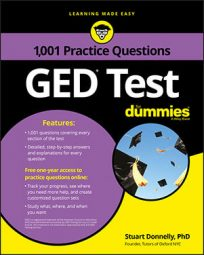Scientific notation makes it easier to work with extremely large or small numbers. Because these kinds of numbers appear with alarming frequency in scientific calculations, you can expect the GED Math exam to include questions that test your ability to work with scientific notation.

## Practice Questions

1. What is the sum of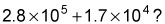(Note: You may not use a calculator.)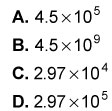2. A human hair has an average diameter of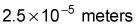What is the maximum number of human hairs that could fit side by side (without overlapping) on a microscope slide that is 50 millimeters wide? Write your answer in scientific notation.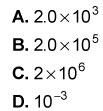1. The correct answer is D.

Rewrite the number with the smaller power of ten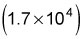so that it has the same power of ten as the larger number: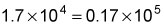Now rewrite the sum using the newly converted number: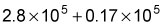Because the powers of ten for the two numbers are now the same, you can simply add the coefficients (the decimal parts) and keep the 105 as it is: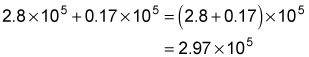Hence, Choice (D) is the correct answer.

2. The correct answer is A.

There are 1,000 millimeters in a meter, so convert the width of the microscope slide from millimeters into meters by dividing by 1,000 (or multiplying by 10–3) to get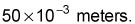Then, to find the number of hairs that can fit on the slide, you need to divide the width of the slide by the width of one hair.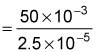Many people make errors entering this on a calculator, so it's usually easier and quicker to perform this calculation by hand. Start by dividing 50 by 2.5 to get 20; then divide the powers of 10 by subtracting the bottom exponent from the top exponent: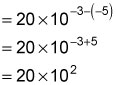Now convert into scientific notation by rewriting 20 as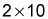(so the leading coefficient is between 1 and 9.9); then multiply the powers of 10 by adding the exponents: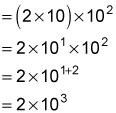Hence, Choice (A) is correct.